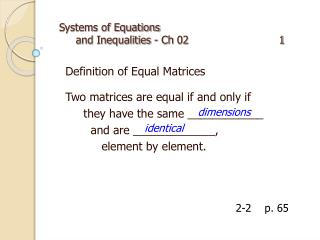Download PresentationSystems of Equations and Inequalities - Ch 02 1Systems of Equations and Inequalities - Ch 02 1 - PowerPoint PPT Presentation

Download PresentationSystems of Equations and Inequalities - Ch 02 1
An Image/Link below is provided (as is) to download presentation

Download Policy: Content on the Website is provided to you AS IS for your information and personal use and may not be sold / licensed / shared on other websites without getting consent from its author. While downloading, if for some reason you are not able to download a presentation, the publisher may have deleted the file from their server.

- - - - - - - - - - - - - - - - - - - - - - - - - - - E N D - - - - - - - - - - - - - - - - - - - - - - - - - - -
Presentation Transcript

1. Systems of Equations and Inequalities - Ch02 1 Definition of Equal Matrices Two matrices are equal if and only if they have the same ____________ and are _____________, element by element. dimensions identical 2-2 p. 65

2. Systems of Equations and Inequalities - Ch02 2 Definition of Addition of Matrices Two sum of two m × n matrices is an m × n matrix in which the elements are the sum of the corresponding elements of the given matrices. 2-2 p. 65

3. Systems of Equations and Inequalities - Ch02 3 Definition of Subtraction of Matrices The difference A – B of two m × n matrices is equal to the sum A + (–B), where –B represents the additive inverse of B. 2-2 p. 66

4. Systems of Equations and Inequalities - Ch02 4 Scalar Product The product of a scalar k and an m×n matrix A is an m×n matrix denoted by kA. Each element of kA is equal to k times the corresponding element of A. 2-2 p. 66

5. Systems of Equations and Inequalities - Ch02 5 Product of Two Matrices The product of an m×nmatrix A and an n×r matrix B is an m×r matrix AB. The ijth element of AB is the sum of the products of the corresponding elements in the ith row of A and the jth column of B. 2-2 p. 67

6. Systems of Equations and Inequalities - Ch02 6 Second-Order Determinant The value of is 2-3 p. 71

7. Systems of Equations and Inequalities - Ch02 7 Third-Order Determinant 2-3 p. 72

8. Systems of Equations and Inequalities - Ch02 8 Identity Matrix under Multiplication The identity matrix of nth order, In , is the square matrix whose elements in the main diagonal, from upper left to lower right, are 1s, while all other elements are 0s. 2-3 p. 72

9. Systems of Equations and Inequalities - Ch02 9 Inverse of a Second-Order Matrix If and then 2-3 p. 72

10. Systems of Equations and Inequalities - Ch02 10 Row Operations on Matrices Interchange any two rows. Replace any row with a nonzero multiple of that row. Replace any row with the sum of that row and a multiple of another row. 2-4 p. 79

11. Systems of Equations and Inequalities - Ch02 11 Vertex Theorem The maximum or minimum value of on a polygonal convex set occurs at a vertex of the polygonal boundary. 2-5 p. 88

12. Systems of Equations and Inequalities - Ch02 12 Linear Programming Procedure Define variables. Write the constraints as a system of inequalities. Graph the system and find the coordinates of the vertices of the polygon formed. Write an expression to be maximized or minimized. Substitute values from the coordinates of the vertices into the expression. Select the greatest or least result. 2-6 p. 91